Algebra Is Magic; A Dialogue

## Algebra Is Magic; A Dialogue

Algebra is boring.

No! Algebra is Magic!

Magic?

Yeah, Magic. You know that Magic isn't really magical'', right? I mean, it's not supernatural. There's always some clever hidden mechanism that makes it work. The illusion is cool, but to me the best part is trying to figure out how they did it! It's like what makes mystery books and movies so interesting, trying to figure out who murdered the butler, and how.

Okay, Mister Magic, so why is algebra magic?

Okay, here's a cool magic trick for you: You know how numbers have two square roots, right?

Sure, like the square root of $4$ is $2$ and $-2$, because multiplying two negatives makes a positive.

Right, well, turns out that there are the same number of roots for any number as the root term. So, two square roots, three cube roots, four fourth roots, and so on, ... fifty two fifty-second roots, ....

Okay....? That's a little weird.

So how do you find them? Like how do you find the three cube roots of 8?

Well, one of them is $2$, right?

Yeah, and the other two?

No idea.

Okay, so here's the trick: The positive root, say, $2$, which is one of the three cube-roots of $8$, is easy to find, and if it wasn't 8, you could find any positive root just using a calculator.

Okay, but you're telling me that there are two more cube roots of $8$...

Negative $2$?

Nope. $-2$ isn't one of them: $-2\times-2\times-2$ is $-8$.

Oh, right. Multiplying by the negative keeps flipping it. So an odd power of a negative number will be negative.

Yes, that's right. There are two more, but they're complex.

Complex like complicated, or complex like the square root of $-1$, that's $i$, right?

The latter ... the $i$ kind of complex.

Yeah, I kinda figured.

So, you know that every number has a complex representation, right?

It's just that the $i$ part, the 'imaginary' part, is usually zero when we do simple math.

Right, so $2$ is the same as $2+0i$, and $-2$ is the same as $-2+0i$.

Right. And you can plot complex numbers on the complex plane, where the first part, the 'real' part is the $X$ axis, and the imaginary part, the 'i' part is the $Y$ axis. Right?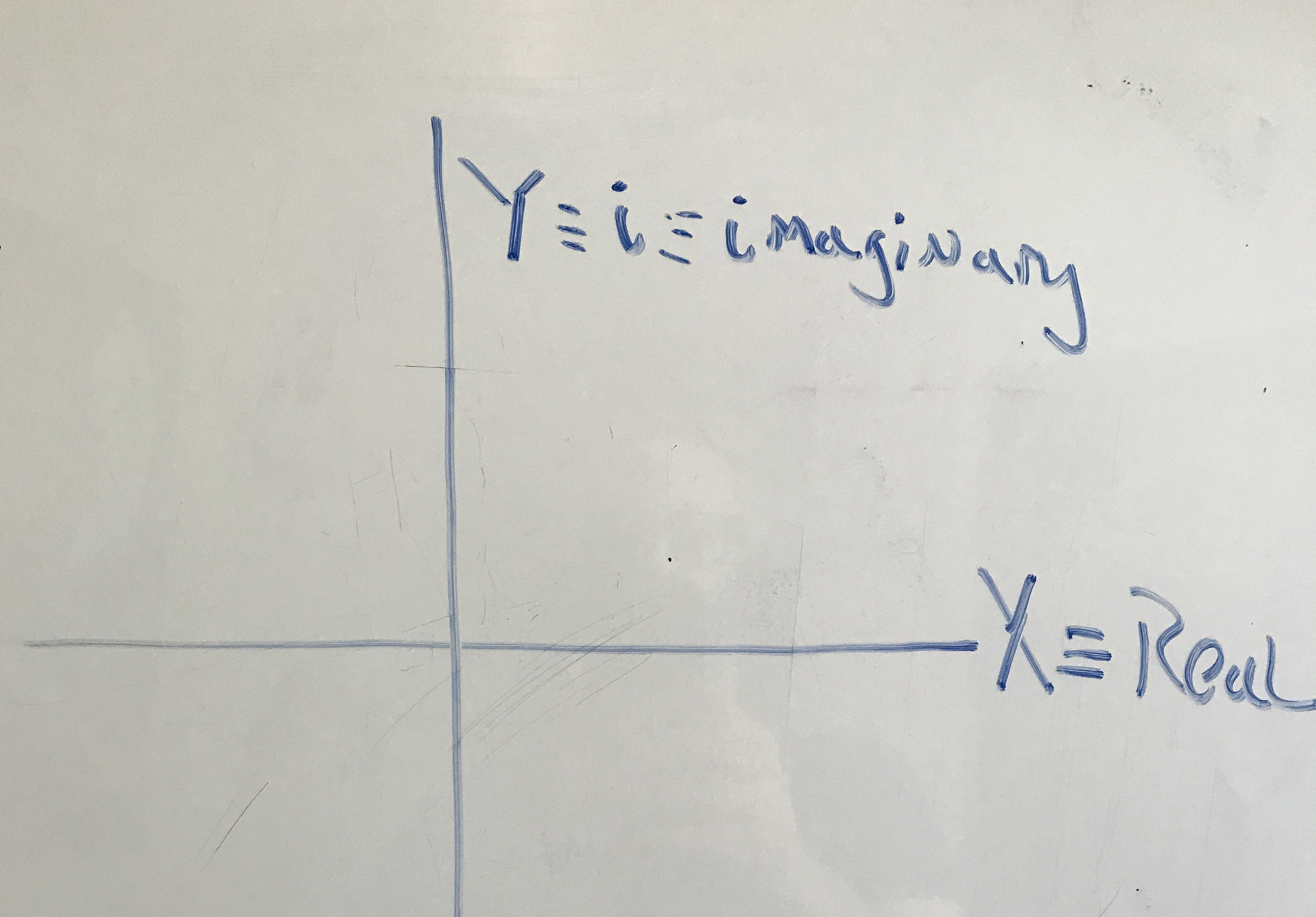Right, okay. I'm with you so far.

Great. So you just need one more thing, which you already know in a slightly different context: You multiple complex numbers just like you multiply polynomials, summing all the cross-products. So, just like $(a+b)\times(c+d)$ is $ac+ad+bc+bd$. So far so good?

I prefer FOIL -- First, Outside, Inside, Last.

Okay, that's fine. It's the same thing, just in a different order.

Okay, great. But I don't see how this review of last year's algebra is going to magically get me the other two cube roots of $8$.

We're about to get to the good stuff right now. First, let's prove that the complex versions of square roots work, just for practice.

Okay.

Let's FOIL out $2$ plus zero $i$ times $2$ plus zero $i$, that is:

$(2+0i)(2+0i)$

By FOIL that's ($2\times2)+(0i\times2)+(2\times0i)+(0i\times0i)$

And all the zeros just zero out, so you're left with $2\times2$ which, conveniently is 4!

Okay, let me try the other side:

$(-2+0i)(-2+0i)$ is $(-2\times-2)+(-2\times0i)+(0i\times-2)+(0i\times0i)$

And again, we're left with $-2\times-2$, which, boringly, is just $4$.

Okay, but that's just cheating. All the imaginary parts just zeroed one another out. So what?

Well, but that's just for square roots. Let's try fourth roots.

Fourth roots? Why not cube roots next?

Fourth roots turn out to be a tiny bit easier for a reason that you'll understand soon.

Okay, fine. Fourth roots, then.

So, what's the fourth root of $16$, that is $\sqrt{16}$?

$2$

Don't forget the negative root.

Oh, okay, $-2$, also right? Because there's an even number of $2$'s and they make a positive. But you're going to tell me that there are two more complex ones, right?

Right. But remember they're all really complex. $2$ is really $2+0i$, and -2 is really $-2+0i$.

Okay, fine. This isn't very magical.

Not yet, but we're building the clever machine that makes the magic work. Let's plot these on our complex plane.

Here's $2+0i$ and $2-0i$.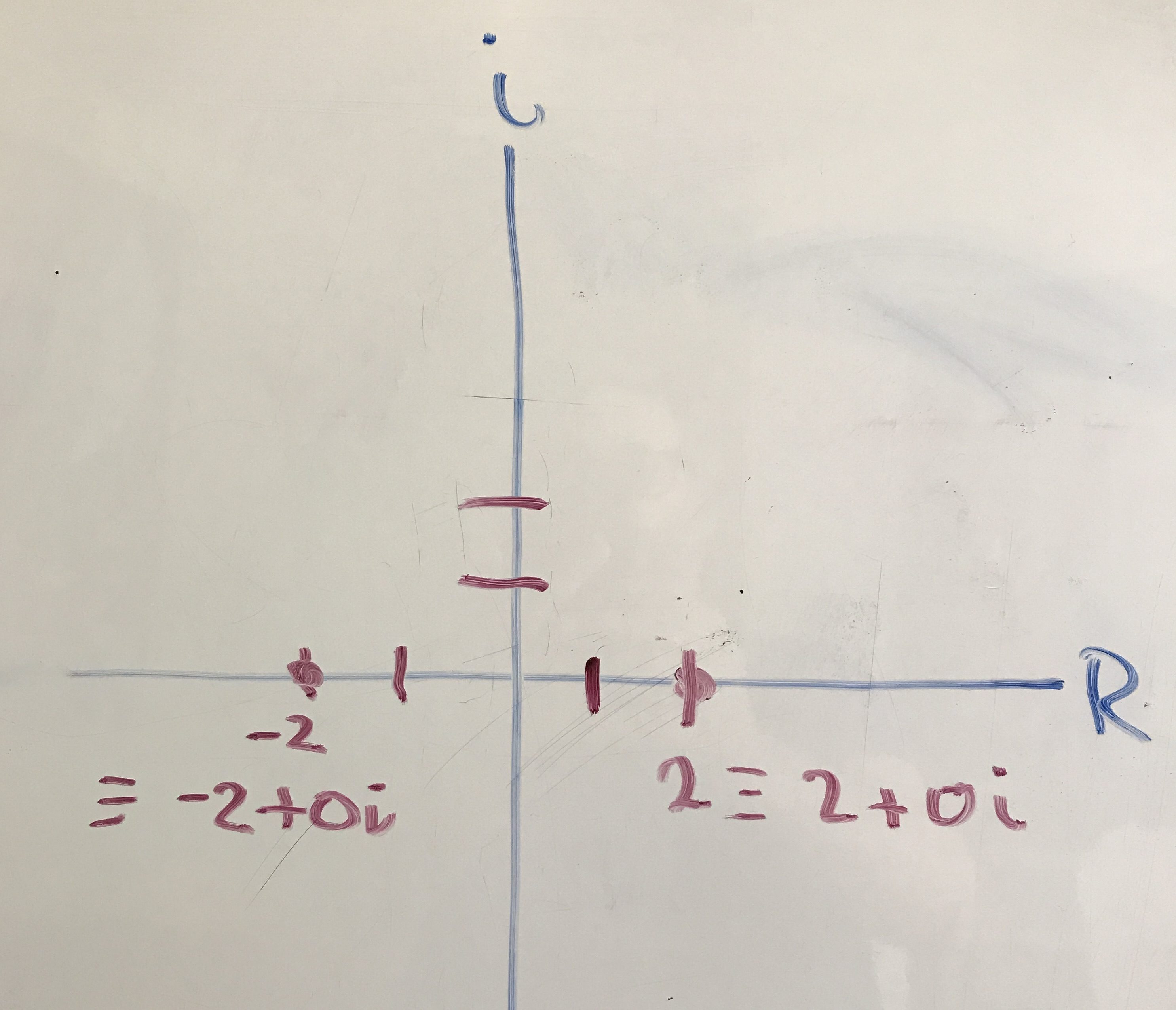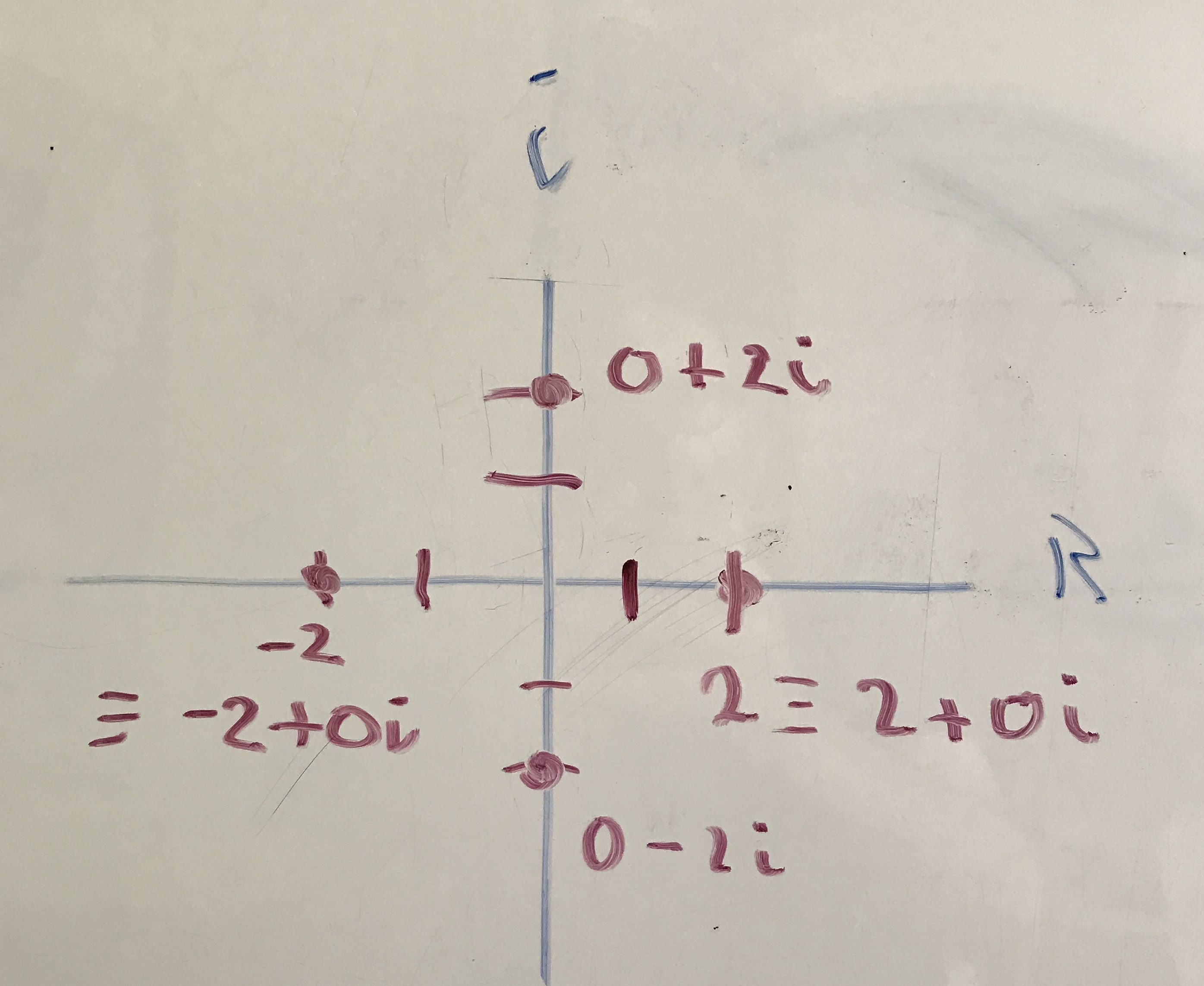Now, here's the core machinery of the magic trick: It turns out that all the roots of a number are evenly spaced around the circle with radius of the positive root of the number. So, the four fourth roots of $16$ are: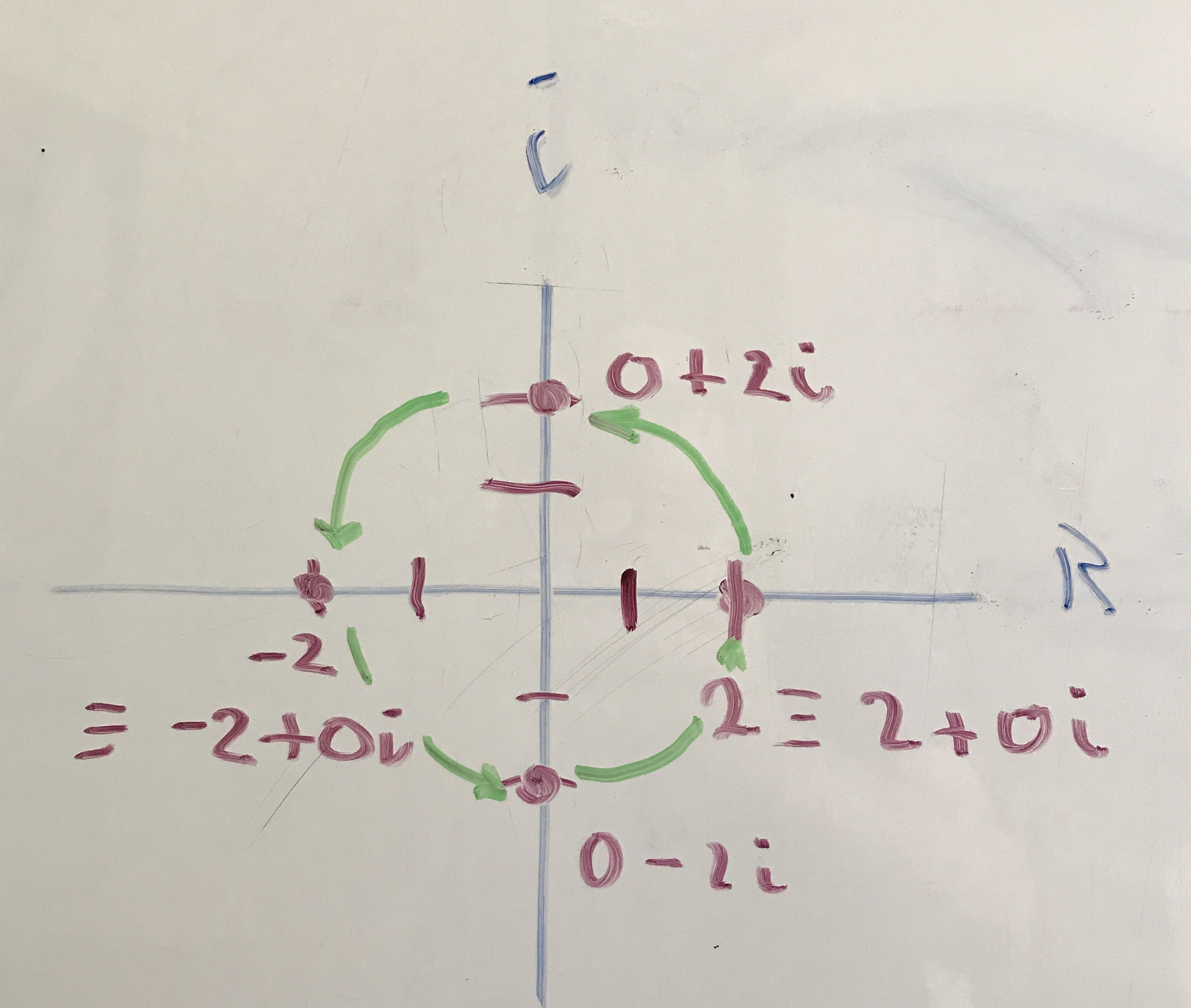So that's $0+2i$ on top, and $0-2i$ on the bottom.

Really?

Yup. Let's prove it by raising $0-2i$ to the fourth power, that is:

$(0-2i)^4$ We could do either one, but $0-2i$ is the less obvious.

Okay. You'll have to watch me through it so I don't make a mistake. That's a lot of FOILs!

We can simplify even roots a bit. You agree that raising $(0-2i)$ to

the fourth power is the same as sqauring it, and then squaring the

result, right?

Sure. It's Like $2^4=(2\times2)\times(2\times2)=4\times4=16$. Right?

Exactly! So go ahead and do the first FOIL of $(0-2i)\times(0-2i)$.

Um, okay, so $0-2i$ squared is $(0-2i)\times(0-2i)$ which by FOIL is

$(0\times0)+(-2i\times 0)+(0\times-2i)+(-2i\times-2i)$. The first

three terms just get zero'ed out, right?

Right, so what's left?

$(-2i)\times(-2i)$, which is$-2i$ squared, that is: $(-2i)^2$.

And what's that? Be careful how you distrbibute the square!

Um, let's see, that's $2$ squared times $i$ squared, or

${(2^2)\times(i^2)}$. $2$ squared is $4$, and $i$ squared is...

... minus $1$ ($-1$)...um, so the result is, um, $4\times-1=-4$. Wait, what happened to the $i$?!

Abracadabra! You just saw some of the magic machinery. The $i$ vanished in a poof of squaring, turning into a $-1$, and flipping the $+4$ to a $-4$.

Oh. Cool.

But we're not done, that's only half the problem.

Oh yeah, we were doing four of them.

Right, but we agreed that you could multiply one pair of them, which you just did, and then multiply those results together again, like we agreed above with all the $2$s.

Hold on, I sort of lost my place. ... Yes, that's right, is was $0-2i$ to the fourth, which is $0-2i$ squared (times itself), and then that result squared (times itself) again.

And we just figured out that $0-2i$ squared is what?

$-4$

And so that squared again is ... ?

Right, $16$...which is what we were looking for!

I'll take your word that the $0+2i$ will do the same thing. Now that I've seen the magical $i^2$ action I can sort of see how that will work out.

Right, so we found the four complex roots of $16$: $2$, $-2$, $0+2i$, and $0-2i$, or, more precisely: $2+0i$, $-2+0i$, $0+2i$, and $0-2i$. And you can see how they are neatly arragned around the circle, evenly spaced, right? Here it is again, because this picture is really important!Right. Cool. I think I can see where we're going next.

Okay, you tell me, then.

Okay, so to get the cube root of something, you divide the circle into three parts instead of four, right?

Exactly! So to make things simple, let's use $2$ again as our starting point. What's $2$ cubed ($2^3$)?

$8$.

Good, so in addition to $2$, we think that there must be two more cube roots of $8$. We know that $-2$ isn't one of them...because the negative would flip it three times. ($-2$ is a cube root of $-8$, but not of $8$.)

Okay. Maybe. I'm not gonna think about the $-8$, and just stick to the cube roots of $+8$ for now.

Good idea. So using the complex plane geometry trick, where should the other roots be?

Um, let's see. So, one of them is $2$, that is, $2+i0$. Let's plot that.Good.

And since there are two more, and they have to be evenly spaced around the circle, they must be here and here. So are they $-2+2i$ and $-2-2i$?

Well, no. If you look at the picture more carefully, the radius of the circle is $2$, but those point aren't 2 on the $X$ and $Y$ axes. Can you figure out what they are? I'll give you a hint: What's $360$ divided by $3$?

$120$. How does that help?

And $120$ minus $90$?

$30$.

So, here, let me draw a right triangle for you.The hypothenuse is the radius -- we've agreed that that's 2, and we know that this angle is $30$ -- that's $120$ less the $90$ from the first quadrant part of the arc, so this angle is $60$. Okay you should be able to take it from here.

Oh, so it's a $30-60-90$ triangle!

Exactly!

I can never remember how they go.

Neither, actually. I'll look it up. [pause] Okay, so if the hypotenues is $2a$, the short leg is $a$ and the long leg is $a$-root-$3$ ($a\sqrt{3}$), that is, $a$ times the square root of $3$.

Ugh. This is going to be a mess!

Not really. Conveniently the hypotenuse is $2$, so $a$ is just $1$. So the short leg is $1$ and the long leg is just the square root of $3$ ($\sqrt{3}$). So what's that point in complex space?

Um. $\sqrt{3}+1i$?

Other way around!

Oh, sorry. $1+\sqrt{3}i$.

Almost; Watch your signs. Use the diagram as a guide. The $1$ is negative and the $\sqrt{3}i$ is positive

Okay, so $-1+\sqrt{3}i$.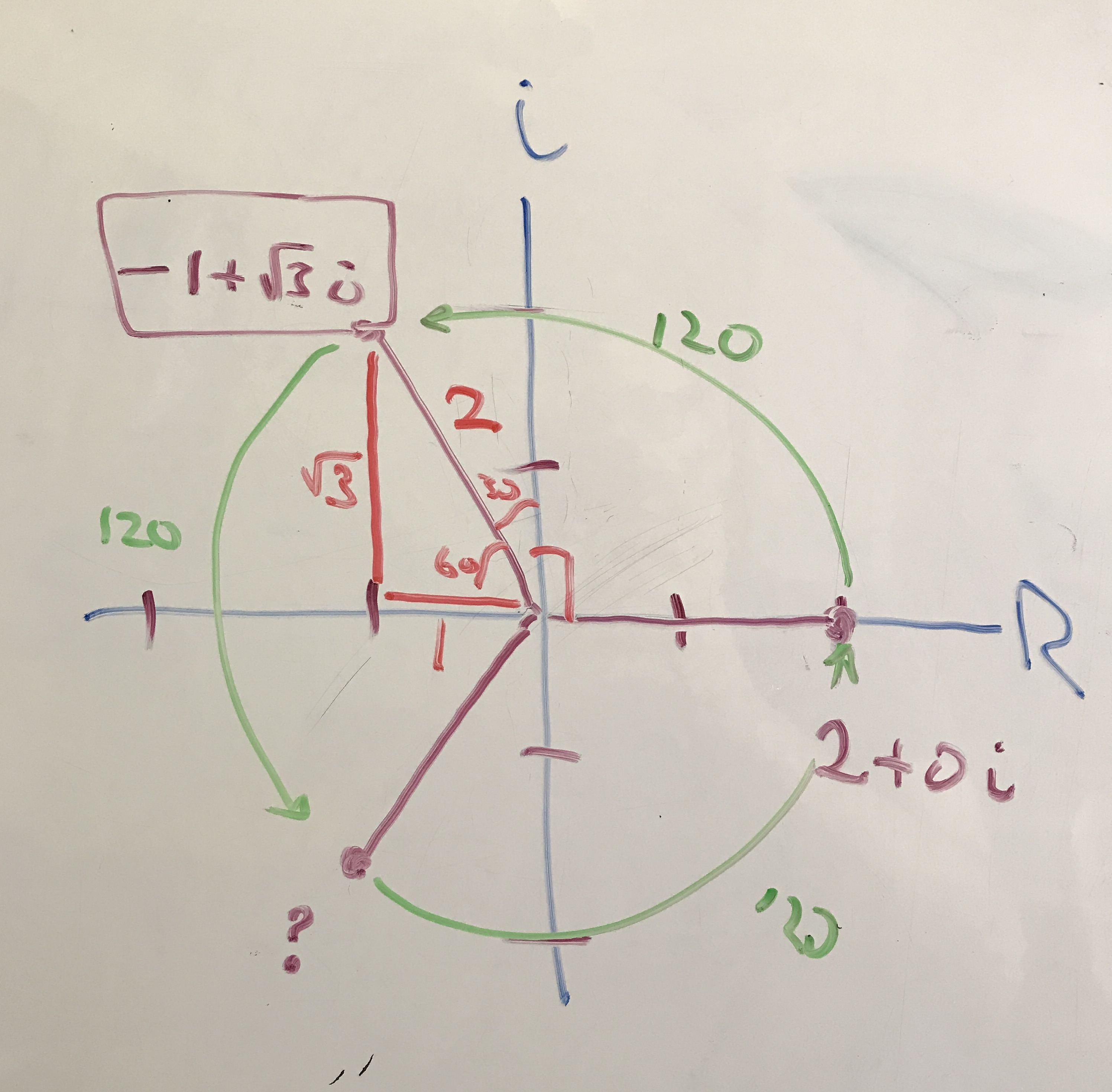Right, so can you prove to yourself that that's a cube root of $8$?

Seriously?! How could something with a square root of $3$ be a cube root of $8$?!

Magic! Let's crank the machine and see.

Okay so...

$(-1+\sqrt{3}i)(-1+\sqrt{3}i)(-1+\sqrt{3}i)$

let's do two of them first: $(-1+\sqrt{3}i)(-1+\sqrt{3}i)$.

FOILing gets us $(-1\times-1) + -\sqrt{3}i + -\sqrt{3}i + (\sqrt{3}i)^2$,

Which is $1 + -2\sqrt{3}i + (\sqrt{3}i)^2$

This seems to be getting worse, not better!

Keep cranking!

Okay so squaring ($\sqrt{3}i$) is $\sqrt{3}\times\sqrt{3}$ which is just 3, and $i\times i$ is $-1$, so that's $-3$ all together, so we have $1+(-2\sqrt{3}i)+-3$, or $-2-2\sqrt{3}i$

Ugh. Now what?

Well, you could just push through the next FOIL multiplcation by ($-1+\sqrt{3}i$), but I suggest factoring out a $2$, and setting it aside for later.

Okay, so that $2$ times ($-1-\sqrt{3}i$) ... almost like the remaning term.

Great, so FOIL those two.

Okay $(-1+\sqrt{3}i)(-1-\sqrt{3}i)$ is $1 -\sqrt{3}i +\sqrt{3}i - (\sqrt{3}i)^2$

Right! And...?

And ... the middle terms cancel out!

Right! And...?

So we have $1 - (\sqrt{3}i)^2$ $\sqrt{3}i$ squared is, again, $3\times-1$, or $-1$ so that's $1-(-3)$...hey, where'd all the root go?!

Magic! Go ahead and finish it.

Okay, so that's $1+3$, which is $4$!

Exactly!

But we were supposed to get $8$!

Oh oh oh ! the $2$ we set aside!

Exactly, so...

So that's $4$ times the factored-out $2$, which is ... $8$!

Holy cow!

Yeah. Magic!

Magic! One way to think about what's happening, is that the complex plane is a machine -- the machine behind the magic trick -- that lets you use geometry to reason about real numbers. When you square a number on the complex plane, you're squaring the real part, but rotating around the plane exactly double the angle from $0$ -- remembering that $0$ is along the positive $X$ axis ... which is the real axis in the complex plane.

I see. Cool!

So, then you should be able to think out the answer to this question...

...okay... (tentatively)

You know that $i$ is the square root of $-1$, right?

Yeah.

So, show that on the complex plane picture.

Oh, well, okay, so is has to be the square root of ... um ... 1?

Yeah.

So that's just $1$, but when I do the square I want it to end up on the left $X$ axis, which is $-1$, or $-1+0i$

Right, so where does it have to be?

Um here?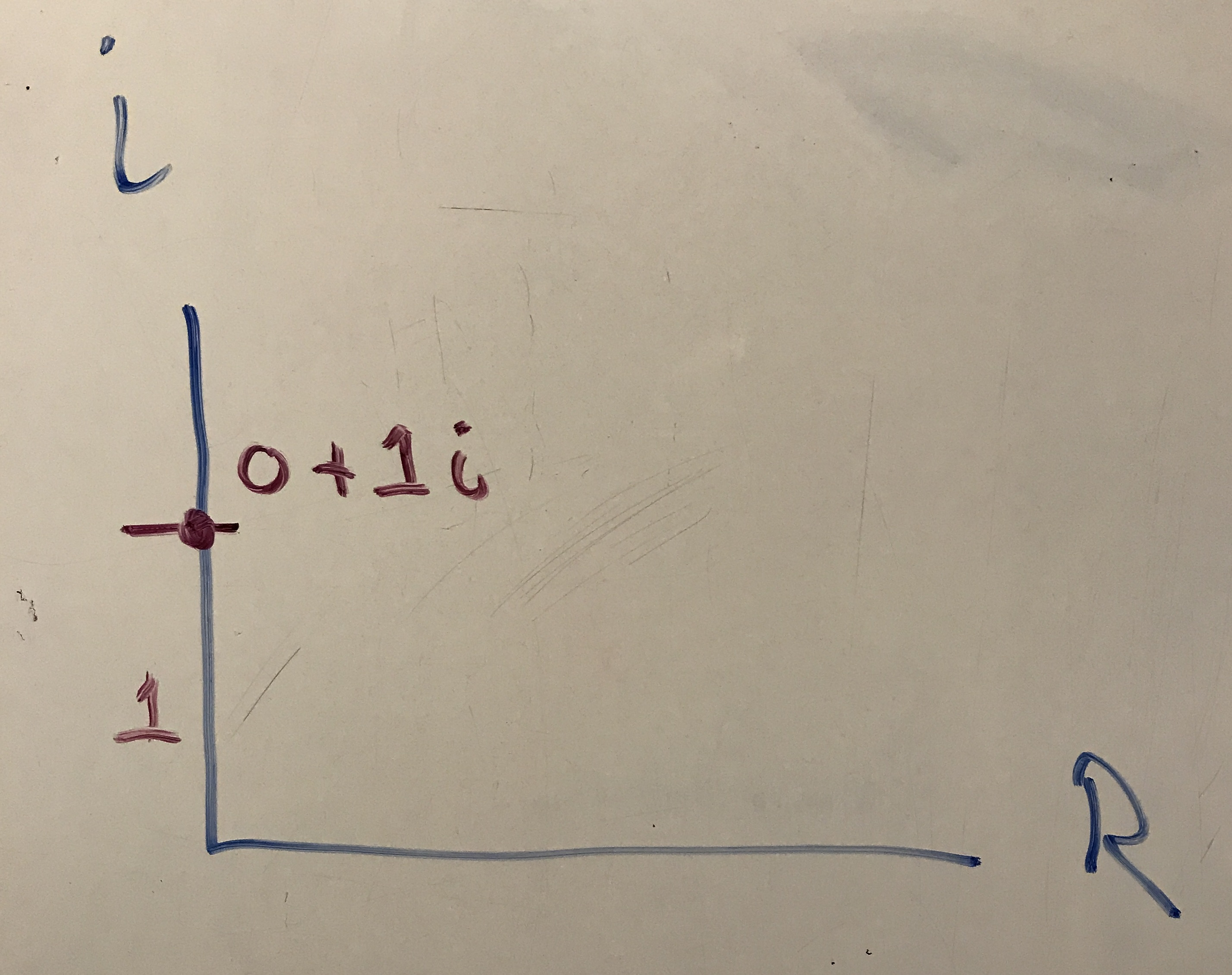Exactly! And what's that point in complex terms?

Oh. I see: $0+1i$.

Right, and since we don't bother writing out zero parts, nor ones, is just ...?

$i$ !!!

Exactly! Okay, here's a slightly harder one that will blow your mind about how great this magic trick ... er, the complex plane is....

Okay....

What's the square root of $i$?

Oh my...Does $i$ have a square root?

Every number has two square roots, including $i$! Just treat it exactly like you did the square root of $-1$. First, where's $i$?

Oh, well, I'm already there. That was just the answer to the last problem: $0+1i$, up here.Right, and where would you have to be for the squaring and rotation rule to work?

Um, so square root of $1$ is just $1$, so the length of the radius will just be one...and it'll be half way between $0+1i$ and $1$, that is $1+0i$, right?Right, so where's that point?

Oh heck, it's someplace in weird root space again, at $45$ degrees!

Yeah, but this one's easy to figure out because the it's just an isosceles right triangle, or, even easier, the sides of a square with diagonal $1$. So what's that point?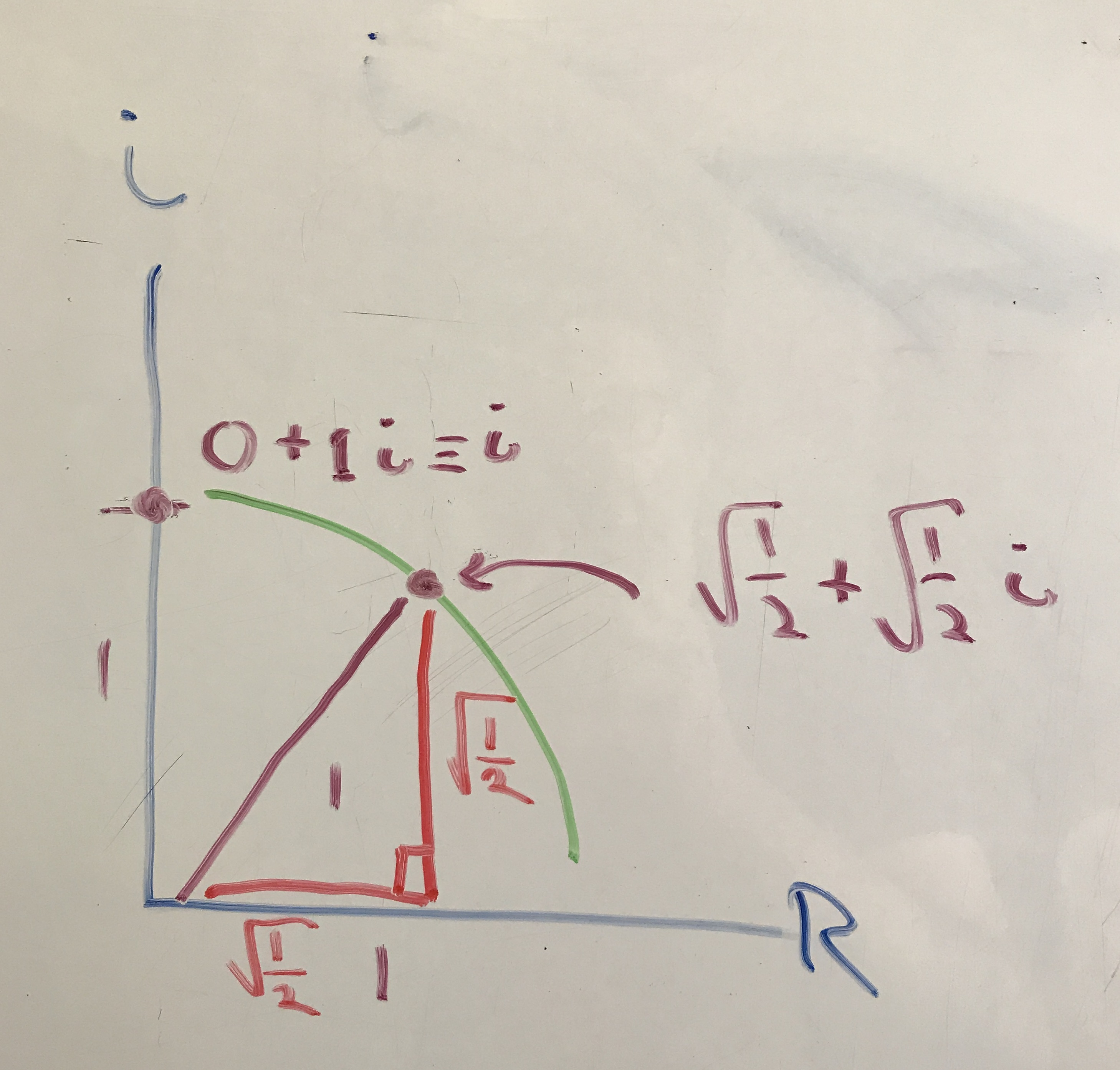Root two in each direction? $\sqrt{2}+\sqrt{2}i$?

Close. That would be if the sides of the square were $1$ and we wanted the diagonal. This is the other way around: The diagonal is $1$, so running the pythagorean theorem backwards you'll see that it's actually the square root of $1/2$, or $\sqrt{1/2}$. So what's that point?

Um, $\sqrt{1/2}+\sqrt{1/2}i?$

Yup! By the way, you'll often see $\sqrt{1/2}$ written as $\sqrt{2}/2$ or as $1/\sqrt{2}$. Someday it's worth proving to yourself that these are all the same thing, but for the moment let's just leave it as $\sqrt{1/2}$.

Ugh. Roots! Is this really going to work?!

Roots are great, especially when you multiply them by themsevles because you get the number back!Also, it turns out that $\sqrt{1/2}$ plays a central role in physics, especially quantum mechanics!

Can we just stick to this problem. Quantum mechanics will make my head hurt.

Fine. Anyway, go ahead and work it out.

Okay, so $({\sqrt{1/2}}+{\sqrt{1/2}i})({\sqrt{1/2}}+{\sqrt{1/2}}i)$ which by FOIL is ($\sqrt{1/2}\times\sqrt{1/2}) + (\sqrt{1/2}\sqrt{1/2}i) + (\sqrt{1/2}\sqrt{1/2}i) + (\sqrt{1/2}i)^2$

This is never gonna work!

Don't bet on it. Just push it through!

Okay, so that's, um, $\sqrt{1/2}$ squared, which is $1/2$

Good! And do the last term first because we're used to those.

Okay, so ${(\sqrt{1/2}i)}^2$ is ${\sqrt{1/2}^2}\times i^2$, which is, um, $(1/2)\times-1, = -1/2$, Oh! So that cancels the first term!

Right, so the real part is just zero. Now do the middle ones.

$(\sqrt{1/2}\sqrt{1/2}i) + (\sqrt{1/2}\sqrt{1/2}i)$

...hmmmm... Well, that's just $2$ times $(\sqrt{1/2}\sqrt{1/2}i)$. That's a little complex. Ha Ha!

Yeah. Ha Ha. I suggest breaking it up into parts.

Okay, so that's $2\times \sqrt{1/2} \times \sqrt{1/2} \times i$. The $\sqrt{1/2} \times \sqrt{1/2}$ is just $(\sqrt{1/2})^2$, which us just $1/2$, and $2\times 1/2$ is just $1$. And that times $i$ is just ...

Holy cow again, it's just $1i$.

To the whole complex result is?

$0+1i$?

Right, which, again is just...

$i$ !!!

See, Magic!

Okay, yeah, Magic! Truly So!

Okay, now remember that every number, including $i$, has two square roots (three cube roots, etc.)! Given what you already know, you can figure out, just by eye, where the other one is.  And you can probably now figure out all the roots of any number pretty easily now. (A little trig, and a calculator will help a lot for figuring out the complex coordinates of weird angles.)

On it!